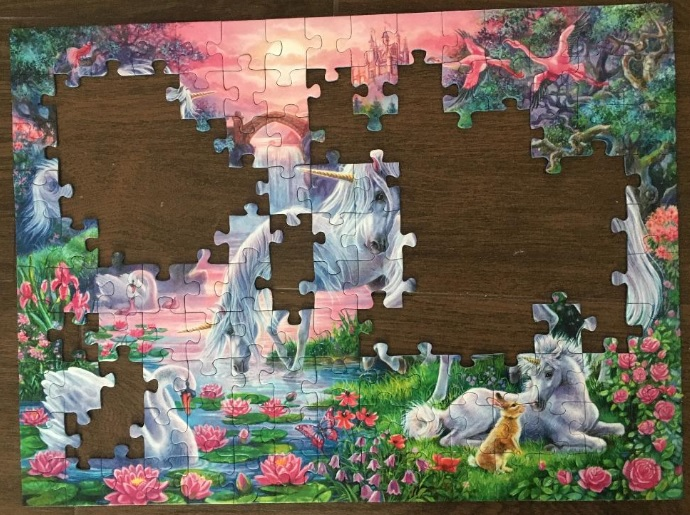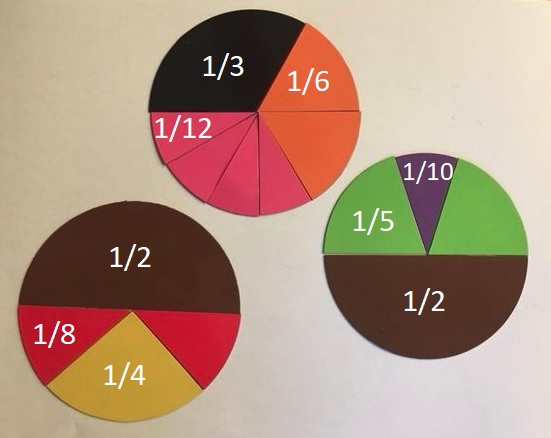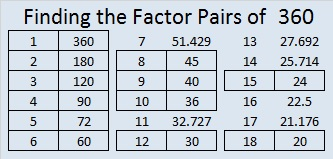# 1491 How Many Square Units Do You See in This Partially Completed Puzzle?

### Today’s Puzzle:

My grandchildren had put this puzzle together many times on their own but wanted me to help them this time. The picture on the box was very helpful and let me know there were four unicorns, but I could only find puzzle pieces for three horns instead of four. I found the missing horn under some furniture in the living room, but I still wondered if any other puzzle pieces had been misplaced. I used multiplication, addition, and subtraction to figure out how many puzzle pieces still needed to be placed on this partially completed puzzle. Can you also figure out the number of puzzle pieces that still need to be placed?I counted the number of puzzle pieces in the box and determined that two pieces were missing. Knowing that two puzzle pieces are missing, can you tell me how many more puzzle pieces are in the box waiting to be placed on the puzzle?

Each puzzle piece is approximately equal to one square unit. If you can determine the area of the entire puzzle and the area of the missing pieces, then you can determine the area of the incomplete puzzle pictured above. Go ahead, give it a try!

### Factors of 1491:

• 1491 is a composite number.
• Prime factorization: 1491 = 3 × 7 × 71.
• 1491 has no exponents greater than 1 in its prime factorization, so √1491 cannot be simplified.
• The exponents in the prime factorization are 1, 1, and 1. Adding one to each exponent and multiplying we get (1 + 1)(1 + 1)(1 + 1) = 2 × 2 × 2 = 8. Therefore 1491 has exactly 8 factors.
• The factors of 1491 are outlined with their factor pair partners in the graphic below.### Another Fact about the number 1491:

1491 is the 21st nonagonal number because
21(7·21 – 5)/2 = 1491.

# 360 What Can You Do With Fraction Circles?

360 has more factors than any previous number. 240 and 336 held the previous record of 20 factors for each of them. How many factors do you think 360 has? Scroll down to the end of the post to find out.

360 can be evenly divided by every number from one to ten except seven, so it was a good number for the ancients to choose when they divided the circle into 360 degrees.I bought a few fraction circles. Each 51 piece set consists of 1 whole circle as well as circles divided into 2 halves, 3 thirds, 4 fourths, 5 fifths, 6 sixths, 8 eighths, 10 tenths, and 12 twelves. What can you do with fraction circles? You can do a lot with them no matter what your age.

Art and Mathematics

The fraction circle shapes can be used just as tangram shapes to create artwork, big or small. A couple of cool symmetric designs can be found at fraction-art and fraction-circle-art. Adding rectangular fraction pieces will increase the possibilities. Here are some simple artistic designs.Fraction Relationships

You can use fraction circle shapes to explore the relationship between fractions such as ½, ¼, and  ⅟₈;  ⅟₃, ⅟₆  and ⅟₁₂; or ½, ⅟₅ and ⅟₁₀:Areas of Parallelograms, Trapezoids, and CirclesThe picture above shows what happens when the circle is divided into four, six, eight, ten or twelve equal wedges, and the wedges are arranged into something that resembles a parallelogram. This idea can be so easily duplicated with these fraction circles without any cutting.

Here are some good questions to ask:

1. What happens to the top and bottom of the shape when the number of wedges increases?
2. Sometimes the resulting shape will look like a trapezoid, and sometimes it looks more like a parallelogram. Why does that happen?We know that the circumference of any circle is 2πr with π defined as the circumference divided by the radius. π is the same value no matter how big or small the circle is.

We can calculate the area of any of the parallelogram-like shapes or trapezoid-like shapes above. Let’s call the length of the bottom of the shape b₁ and the length of the top b₂. The area of the resulting shape is calculated: A = ½ · (b₁ + b₂) · h. Since b₁ + b₂ = 2πr, and the height equals the radius, we can write our formula for the area of a circle as A = ½ · 2πr · r = πr².

This exercise demonstrates that the area of rectangles, parallelograms, trapezoids, and circles are all related!

Introduction to Pie Charts

Pie charts are a great way to display data when we want to look at percentages of a whole. If you use fraction circles, you are limited to using only to certain percentages, but they can still make a good introduction to the subject. To make the pie chart work either the total of all the degrees will have to equal 360 or the total of all the percents will have to equal 100:After a brief introduction using the fraction circles, try Kids Zone Create a Graph. It’s really easy to use!

Exploring Perimeter and Introducing Radians in Trigonometry

The perimeter of each fraction circle piece can be calculated. If the r = 1, the circumference of the circle is 2π, and we can see an important relationship between the degrees and the perimeter of each piece.What experiences have YOU had with circle fractions? Did you find them frustrating or enlightening? Personally, I like them very much, but I wish they had also been cut into ninths.

Here are some facts about the number 360:

The interior angles of every convex or concave quadrilateral total 360 degrees.

The exterior angles of every convex or concave polygon also total 360 degrees.

Here is all the factoring information about 360:

• 360 is a composite number.
• Prime factorization: 360 = 2 x 2 x 2 x 3 x 3 x 5, which can be written 360 = 2³·3²·5
• The exponents in the prime factorization are 3, 2 and 1. Adding one to each and multiplying we get (3 + 1)(2 + 1)(1 + 1) = 4 x 3 x 2 = 24. Therefore 360 has exactly 24 factors.
• Factors of 360: 1, 2, 3, 4, 5, 6, 8, 9, 10, 12, 15, 18, 20, 24, 30, 36, 40, 45, 60, 72, 90, 120, 180, 360
• Factor pairs: 360 = 1 x 360, 2 x 180, 3 x 120, 4 x 90, 5 x 72, 6 x 60, 8 x 45, 9 x 40, 10 x 36, 12 x 30, 15 x 24 or 18 x 20
• Taking the factor pair with the largest square number factor, we get √360 = (√10)(√36) = 6√10 ≈ 18.974